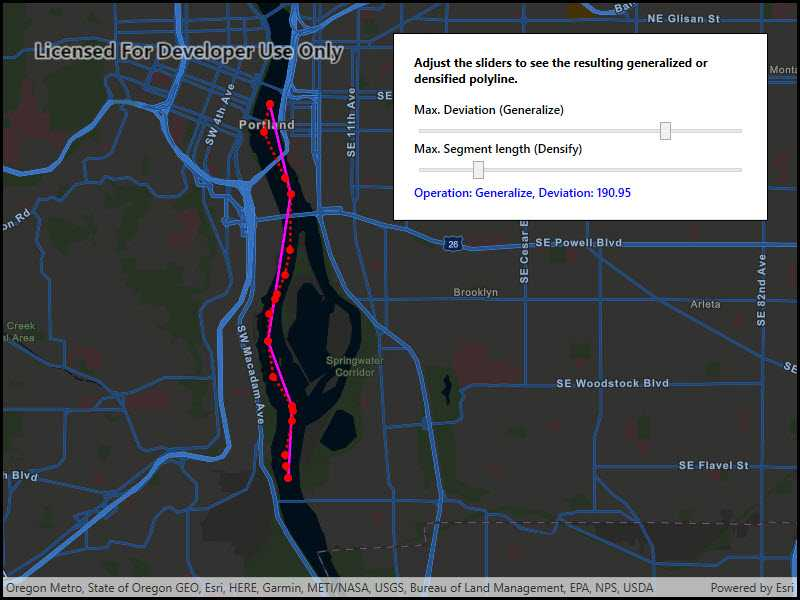# Densify and generalize

View inWPFUWPFormsiOSAndroid
View on GitHub
Sample viewer app

A multipart geometry can be densified by adding interpolated points at regular intervals. Generalizing multipart geometry simplifies it while preserving its general shape. Densifying a multipart geometry adds more vertices at regular intervals.## Use case

The sample shows a polyline representing a ship's location at irregular intervals. The density of vertices along the ship's route is appropriate to represent the path of the ship at the sample map view's initial scale. However, that level of detail may be too great if you wanted to show a polyline of the ship's movement down the whole of the Willamette river. Then, you might consider generalizing the polyline to still faithfully represent the ship's passage on the river without having an overly complicated geometry.

Densifying a multipart geometry can be used to more accurately represent curved lines or to add more regularity to the vertices making up a multipart geometry.

## How to use the sample

Use the sliders to control the parameters of the densify and generalize methods.

## How it works

1. Use the static method `GeometryEngine.Densify(polyline, maxSegmentLength)` to densify the polyline object. The resulting polyline object will have more points along the line, so that there are no points greater than `maxSegmentLength` from the next point.
2. Use the static method `GeometryEngine.Generalize(polyline, maxDeviation, true)` to generalize the polyline object. The resulting polyline object will have points shifted from the original line to simplify the shape. None of these points can deviate farther from the original line than `maxDeviation`. The last parameter, `removeDegenerateParts`, will clean up extraneous parts of a multipart geometry. This will have no effect in this sample as the polyline does not contain extraneous parts.
3. Note that `maxSegmentLength` and `maxDeviation` are in the units of the geometry's coordinate system. In this example, a cartesian coordinate system is used and at a small enough scale that geodesic distances are not required.

## Relevant API

• GeometryEngine
• Multipoint
• Point
• PointCollection
• Polyline
• SimpleLineSymbol
• SpatialReference

## Tags

data, densify, generalize, simplify

## Sample Code

DensifyAndGeneralize.xamlDensifyAndGeneralize.xaml.cs

``````﻿<UserControl x:Class="ArcGISRuntime.WPF.Samples.DensifyAndGeneralize.DensifyAndGeneralize"
xmlns="http://schemas.microsoft.com/winfx/2006/xaml/presentation"
xmlns:x="http://schemas.microsoft.com/winfx/2006/xaml"
xmlns:esri="http://schemas.esri.com/arcgis/runtime/2013">
<Grid>
<esri:MapView x:Name="MyMapView" />
<Border Style="{StaticResource BorderStyle}">
<Grid>
<Grid.RowDefinitions>
<RowDefinition Height="Auto" />
<RowDefinition Height="Auto" />
<RowDefinition Height="Auto" />
<RowDefinition Height="Auto" />
<RowDefinition Height="Auto" />
<RowDefinition Height="Auto" />
</Grid.RowDefinitions>
<TextBlock Text="Adjust the sliders to see the resulting generalized or densified polyline."
Grid.Row="0" Margin="0,0,0,15"
TextWrapping="Wrap" FontWeight="Bold" />
<TextBlock Text="Max. Deviation (Generalize)"
Grid.Row="1"
FontWeight="SemiBold" />
<Slider x:Name="DeviationSlider"
Grid.Row="2" Margin="0,5,0,0"
Value="10" Minimum="1" Maximum="250" />
<TextBlock Text="Max. Segment length (Densify)"
Grid.Row="3"
FontWeight="SemiBold" />
<Slider x:Name="SegmentLengthSlider"
Grid.Row="4" Margin="0,5,0,5"
Value="100" Minimum="100" Maximum="500" />
<TextBlock x:Name="ResultLabel"
Grid.Row="5"